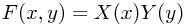As mentioned to the side, this article will discuss everything from the relevance of Stone-Weierstrass to practical aspects of solving differential equations.

For now though, there's just this cute observation about a condition for a nice smooth function to be multiplicatively separable:

A (twice continuously differentiable) function F(x,y) (away from areas where it or its derivatives are zero) can be decomposed as F(x,y) = X(x) Y(y) if and only if it satisfies the differential equation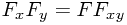This obviously holds if the function is separable in this way: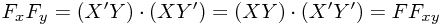The other way was slightly surprising the first time I noticed it (trying to simplify a GR metric, in fact):

First, rearrange the equation as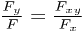And then observe that this takes the form of the y derivative of logarithms: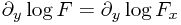which can easily be integrated to give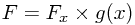for some function g(x) coming from the 'constant' of integration (which was with respect to y). But now we can rearrange and integrate once more to find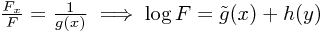from which we obtain